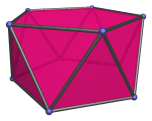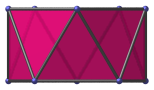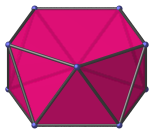# The Pentagonal Antiprism

The pentagonal antiprism is a 3D uniform polyhedron bounded by 12 polygons (2 pentagons and 10 triangles), 20 edges, and 10 vertices.The pentagonal antiprism can be derived from the regular icosahedron by cutting off two opposite vertices. Conversely, we can erect two pentagonal pyramids on the pentagonal antiprism, one on each of its pentagonal faces, and thus obtain the icosahedron.

## Projections

In order to be able to identify the pentagonal antiprism in various projections of 4D objects, it is useful to know how it appears from various viewpoints. The following are some of the commonly-encountered views:

Projection Envelope DescriptionRegular decagon

Parallel projection centered on a pentagonal face.Rectangle

Parallel projection parallel to pentagonal faces.Parallelogram

Parallel projection centered on an edge shared by two triangular faces.Octagon

Vertex-centered parallel projection.

## Coordinates

The Cartesian coordinates of the pentagonal antiprism, centered on the origin and having edge length 2, are:

• ±(√(2(5+√5)/5), 0, √((5+√5)/10))
• ±(√((5−√5)/10), ±φ, √((5+√5)/10))
• ±(√((5+2√5)/5), ±1, −√((5+√5)/10))

where φ=(1+√5)/2 is the Golden Ratio.

These coordinates give a pentagonal antiprism parallel to the XY plane, in a “nice” orientation for placing in 3D scenes.

An alternative set of coordinates can be obtained by exploiting the pentagonal antiprism's connection with the icosahedron. Removing two opposite vertices from the icosahedron and taking the convex hull of the remaining 10 vertices yields the pentagonal antiprism. Taking the origin-centered coordinates for the icosahedron as our starting point, we obtain the following origin-centered coordinates for the pentagonal antiprism:

• ±(0, 1, −φ)
• (±1, ±φ, 0)
• (±φ, 0, ±1)

These coordinates do not yield a nice orientation of the pentagonal antiprism, but they are algebraically nicer in that all coordinates can be expressed in terms of multiples of the Golden Ratio, and do not involve nested radicals.

## Occurrences

The pentagonal antiprism occurs as cells in the grand antiprism.

It also occurs as cells in the following CRF polychora:

Last updated 17 Jun 2019.